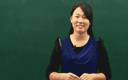### 四年级英语上册Module 4 Unit 11《Shapes》（Period 2）

• 同步课程
• 本节重点
• 主讲老师

《Shapes》（Period 2）
Look and say

What shapes can you see?
circle     This is a circle.
star       This is a star.
rectangle  This is a rectangle.
triangle   This is a triangle.
square     This is a square.

Let's learn
oval      This is an oval.
diamond   This is a diamond.
heart     This is a heart.

Ask and answer
How many stars are there?
circles
squares
triangles
rectangles
S1:How many stars are there?
S2:There are eight stars.
S1:How many circles are there?
S2:There are eight circles.
S1:How many squares are there?
S2:There are three squares.
S1:How many triangles are there?
S2:There are nine triangles.
S1:How many rectangles are there?
S2:There are ten rectangles.

Draw and say
This is a circle.I can draw an orange in it.
This is a square.I can draw a clock in it.
This is a triangle.I can draw a biscuit in it.
This is a rectangle.I can draw a biscuit in it.
This is a star.I can draw a starfish in it.

Let's check
picture A
shape      star   rectangle   circle   square   triangle
How many   1      2           7        5        5
picture B
shape      star   rectangle   circle   square   triangle
How many   2      5           3        6        1

Look,count and write
1.There are three triangles.
2.There are four circles.
3.--How many squares are there?
--There are seven squares.
4.--How many stars are there?
--There are five stars.
5.--How many rectangles are there?
--There are nine rectangles.

Read,choose and write
Peter:We're home,mum.
Miss Brown:How are you today?
Peter:We're thiiiirsty and hungry.
Miss Brown:Have some cakes and orange juice.
Peter&Paul:Thank you,Mum.

Peter:This cake is big.It's for you,Paul.
Paul:Thank you,Peter.Look,It's a star,How nice!
Peter:Taste it.How is it?
Paul:It's sweet and a little(一点)sour.
It's a lemon and cake.
Peter: My cake is a rectangle.
It's a chololate cake.It's sweet!

Read and choose
I can draw pictures very well.Look,this is a monster,It has a head,It is a circle.Its eyes are triangles.Its ears are squares.Its body is a square.The monster has four long legs.Its has four feet.They are triangles.©2016 同桌100 All Rights Reserved